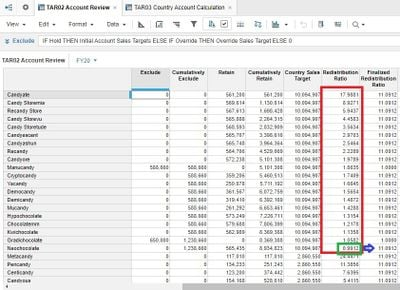# Moving numbers from one column to the nextHi please refer screenshot below. I am trying to move the last number in the column highlighted in red (Redistribution Ratio) to the next column, Finalized Redistribution Ratio. There are 20 rows in the highlighted part of the column. Redistribution ratios will automatically recalculate for each country, formula already working. The number to be copied to the adjacent column is 0.9912 which appears on the last row of the highlighted box in red. The Finalized Redistribution Ratio column should populate in such a way the number to be copied over i.e. 0.9912 appears 20x corresponding to each row in the highlighted box to its left.

Question is how does one do this? Am thinking solution would be to have a number column in the module that ranges from 1 to N where N is the number of rows in the module. As each number is unique we should then be able to use it as an index for a lookup as the intersection of that number and the Redistribution Ratio column would be the number we're looking for and then we would just move that number to the column on its right, the Finalized Redistribution Ratio column. Of course we would then have to populate it in the manner described above.

I know this sounds a bit complicated but there must be a solution for this so appreciate your ideas!Kind regards,

Clarence

Tagged:

•There might be better solutions but I finally managed to solve by taking the following actions:

1. Creating a SYS module with a Rank Flat list as dimension containing all the Redistribution ratios in the module;

2.  Setting up the lookups I needed within the TAR03 module;

3. Also within the TAR03 module looking up the Redistribution ratio in the SYS module referred in 1. above.

Correct me if wrong but what I learnt was for the lookup to work I first needed to convert numeric row references / indices to a ranked list format and then using the list format to lookup the ratio needed in the SYS module.

Kind regards,

Clarence

•You are indeed correct in that a LOOKUP will only work when the lookup line item is a list formatted item.

However, you could use MIN summary method and then SELECT to reference the top level of the product list.

This may have been a simpler solution!

•